×
Log in to StudySoup
Get Full Access to C of C - MATH 111 - Study Guide - Midterm
Join StudySoup for FREE
Get Full Access to C of C - MATH 111 - Study Guide - Midterm

Already have an account? Login here
×
Reset your password

# dr lavrich Description

##### Uploaded: 11/18/2016
45 Pages 152 Views 0 Unlocks
Reviews

1 Note Taker= Lacy Jones Professor/ institution=Dr. Lavrich-CofC Book= Atoms First 2nd edition by Julia Burdge and Jason Overby Chem 111Test III Study Guide Covers chapters 7 8 & 9 Key: * = This means that Dr. Lavrich Stressed this during class, or the book made note of it. OR *=excited state → This arrow means furthering information of the statement () This means that there is an AKA form or side note, or there is a source. My notes contain images from sources other than the Atoms First book, so I have to cite. This color means book topic Headlines This color is for definitions This color is for example problems This color with a high light is the answer2 This color means that the notes come from lecture and what day This color means Lecture Headlines This color emphasizes such as valence electrons This color is for the very end of the notes pages and covers key tables the professor wants us to memorize, constants and Equations, and also difficult concepts. = 1s^2 fully occupied = 1s^1 half occupied --------------------------------------------------------------------------------------------------------------------- Chapter 7 Bonding Theory and Molecular Geometry (3d Shape) 1. Valence Shell e- pair repulsion (VSEPR) AKA Lewis Theory~ -E- pairs repel one another - Non bonding (lone pair) -Shared pair (Bonds)  : H-O-H  : E- Domain: Pair of e Ex. CO2  : :  O=C=O  : : 2 e- Domains, no matter what the bond type is it counts as a single domain. : : :3 O=O-O: : : 3 Domains, → 2 bonds (single double) 1 lone pair Arrangement of e- domains~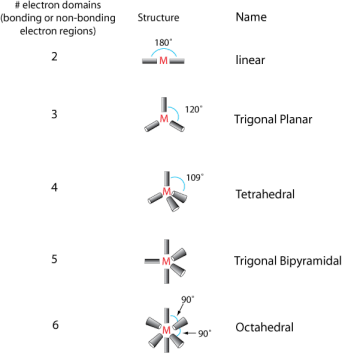For Trigonal Bipyramidal There is axial bonds by 90 degrees, and Equatorial bonds by 120 degrees4Procedure for these arrangements~ 1. Draw Lewis Structure 2. Determine the number of e- domains on central atom 3. Determine the electron domain geometry with VSEPR → Lone pair be as far away as possible 4. Determine the molecular geometry → Based on the positions of the bonded atoms, not on lone pairs (Neglecting them) Ex: System Examples # of domains E- Domain Geometry Molecular Geometry AB3 3 Trigonal Planar Trigonal Planar AB2 1 lone pair 3 Trigonal Planar Bent (Because linear but another electron domain from the lone pair pushes the bonds down) AB4 4 Tetrahedral Tetrahedral (AB3) 3 bonds, 1 lone pair 4 Tetrahedral Trigonal Pyramid. Angles are Less than 109.5 (AB2) 2 bonds, 2 lone pairs 4 Tetrahedral Bent

## 60 moles of CO(g) reacts with excess of O2, how much can be produced?If you want to learn more check out .0821 constant

5 AB5 all bonded 5 Trigonal Bipyramid Trigonal Bipyramid AB4 4 bonded, 1 lone pair 5 Trigonal Bipyramid See-saw AB3 3 Bonds and 2 lone Pairs 5 Trigonal Bipyramid T-Shape AB2 2 bonds 3 lone pairs 5 Trigonal Bipyramid Linear AB6 all bonded 6 Octahedral Octahedral AB5 5 bonds 1 lone pair 6 Octahedral Square Pyramid AB4 4 bonds 2 lone pairs 6 Octahedral Square Planar

* Best orientation is when the bonds farthest away from lone pairs.6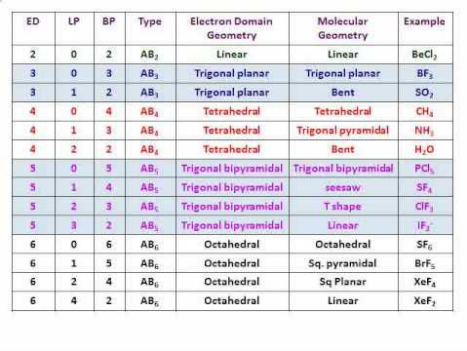Ex 1: BH3 NH3 NH4+ Valence: 6 8 8 Lewis Structure: Domains: 3 4 4 Geometry: Trigonal (TD) (TD) Planar Ex 2: # of domains: 3 SO3 (24e-)7 Trigonal planar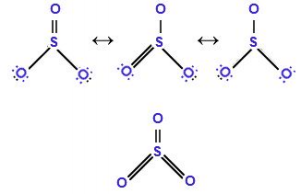SO2(18e-) Bent (Presence of lone pairs)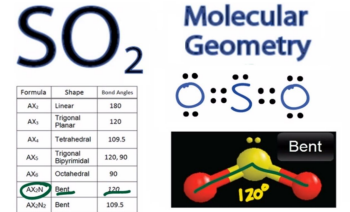CH4 Tetrahedral8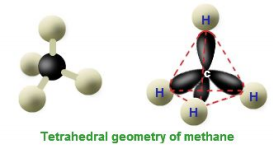NH3 Trigonal pyramid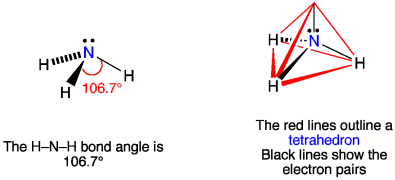H2O Bent9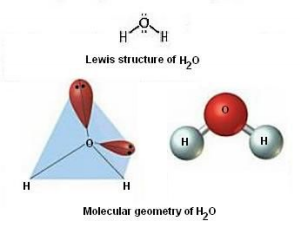PF5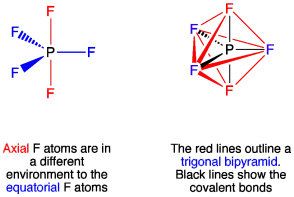SF410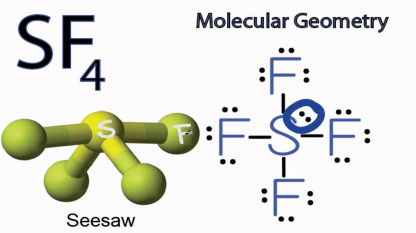ClF3XeF211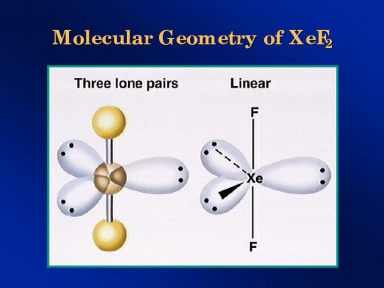# of Domains 6 SF6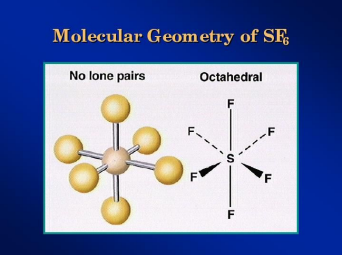12 BrF5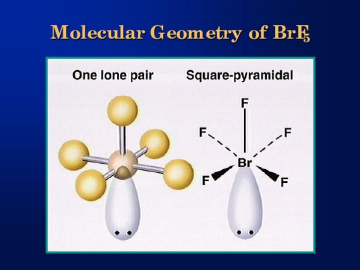ClF4-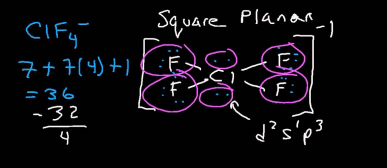Multiple Central Atoms: -Consider # of domains around each central atom CH3OH13 c= 4 domains }Tetrahedral for both O= 4 domains Later the O becomes bent Deviations from Ideal Angles: -NH3 => Tetrahedral14 It reduces the angles from 109.5 -CH2O Pushes to 110 degree, making more room for themselves 7.6: VSEPR15 a. CH3I 4 domains → Tetrahedral B. ClF316 5 domains → T shaped C. H2S17 4 domains → Bent Molecular Geometry & Polarity: Molecular Geometry:Shape Polarity: Chemical/ Physical Property CO2 H2O O=C=O H/O\H -Non Polar Polar -2 dipole moments -2 dipole moments don’t cancel out because of Z Equal but opposite That cancel each other out18 Limitations of this Theory: -Describes covalent bond in the same way Eg. Single Bond→ Shared pair of e Valence bond Theory: -Retains Lewis Concept of atoms sharing e- ↳add quantum #’s (n, l, ml) Covalent Bond: Overlap of singly occupied atomic orbitals, one on each atom of a bonding pair H2 → H-H H H 1s^1 1s^1 Partly shaded Partly shaded Overlap and electrons fill overlap HF Two Important Aspects of VB vs. VSPER 1. Explains why covalent bonds form in the first place19 ↑ Both graphs represent the same relationship I. Large r (r>re)~ Isolated atoms, NO interaction Ii. r→ re ~ Decrease in potential energy Iii. r=re~ Formation of covalent bond IV. r<re ~ Repulsion dominate Rocks back and for→ Constant movement 2) Explain the directionality of the bond → How it is orientated in space H2S20 H-S-H Sulfur: [Ne] 3s^2 3p^4 H: 1s^1 90 degrees between H2 Valence Bond Theory Limitations~ BeCl2 BF3 }All stable, and exist, but can’t be explained by this theory CH4 BeCl2 Be: 1s^2 2s^2 _ _ _ 2p _↓↑ 2s _↓↑ 1s The Be doesn’t have a lone pair for the Cl BF3 1s^2 2s^2 2p^1 ↑_ _ _ 2p _↓↑ 2s _↓↑ 1s CH4 1s^2 2s^2 2p^221 ↑_ ↑ _ _ 2p _↓↑ 2s _↓↑ 1s Hybridization of atomic orbitals~ -Used to create sufficient number of singly occupied orbitals Process 1. Promotion of e 2. Mixing of orbitals containing unpaired e- → This is to create the same type of orbital. Called atomic hybrid, because localized on a particular element ↑ Keeps the VB theory alive, because this is completely man made BeCl2 Be: 1s^2 2s^2 _ _ _ 2p _↓↑ 2s _↓↑ 1s 1) Promotion, Be* (*=excited state) _↑ _ _ 2p _↑ 2s _↓↑ 1s 2 singly occupied, 2 bonds. Be * ↓↑_ ↑_ ↑_ _ _ → ↑_ ↑_ _ _  1s 2s 2p sp atomic Hybrid PCl522 P: _ _ _ _ _ 3d ↑_ _ _ _ _ ↑_ ↑_ ↑_ 3p → ↑ _ ↑_ ↑_ ↓↑_ 3s ↑_ ↑_↑_↑_↑_↑_ sp3d hybrid SF6 _ _ _ _ _ 3d ↑_ ↑_ _ _ _ ↓↑_ ↑_ ↑_ 3p → ↑_ ↑_ ↑_ ↓↑_ 3s ↑_  (Promote)→ ↑_↑_↑_↑_↑_ _ _ Sp3d2 hybrid Unhybridized d-atomic Hybrid Orbitals: 1. Form first bond between two atoms 2. Hole lone pairs ↪Multiple bonds → Used on hybridized atomics. Overlap23 NH3 -Need 4 hybrids ↪ 3 singly occupied N-H -1 lone pair N: 1s^2 2s^2 2p^3 ↑_ ↑_ ↑_ 2p ↑↓_ 2s No need for promotion just mixing ↑↓_↑_↑_ _ Sp3 hybrid The double arrow is the line pair, and single is the N-H ICl3 -Need 5 hybrids of I -3 singly occupied I-Cl -2 doubly occupied lone pairs I: 5s^2 5p^5 _ _ _ _ _ 5d ↑_ _ _ _ _ ↑↓_ ↑↓_ ↑_ 5p → ↑↓_ ↑_ ↑_ ↑↓_ 5s ↑↓ _24 ↑↓_ ↑↓_ ↑_ ↑_↑ _ _ _ _ _ _ sp^3 d d-atomics Multiple Bonds: (double triple) -involve left over unhybridized atomic CH2O -C needs 3 singly occupied orbitals for each C-H bond, 1 singly occupied atomic -O needs 3 hybrids: 1 for C-O 2 for lone pairs, 1 singly occupied atomic C ↑_ ↑_ _ 2p → ↑_ ↑_ ↑_ ↑↓_ 2s ↑_ ↑_ ↑_ ↑_ ↑_ sp^2 hybrid p(unhybridized) Carbon: Sp^2 hybridized O ↑↓_ ↑_ _ 2p → _ _ _ ↑↓_ 2s _ Promotion not necessary ↑↓_ ↑↓_↑ _ ↑_ sp^2 hybrids p atomic singly occupied CH2Cl225 E- domain: tetrahedral Molecular geometry: tetrahedral Bond angles: 109.5 Polar: yes C: ↑_ ↑_ _ 2p ↑↓_ 2s ↑_↑ _↑ _ ↑_ sp^3 NCLO: -N is central N: -needs 3 hybrids (1 for N-C bond, 1 for N-O bond, 1 for lone pair) -1 unhybridized atomic (double bond)26 ↑_ ↑_ ↑_ 2p ↑↓_ 2s ↑↓_↑ _ ↑_ ↑_ Sp^2 hybrids P atomic E- domain geometry: 3 domains → trigonal planar Molecular geometry: 1 lone pair → bent Angles: <120 → Because of lone pair Polarity: polar molecule SP^2 hybrid Te Cl2 Br2 E- domain: trigonal bipyramid Molecular geometry: see-saw Bond angles <90, <120 Te needs: -5 hybrids (lone pair for 1, 4 bonds) Te: _ _ _ _ _ 5d ↑ _ _ _ _ _ ↑↓_ ↑_ ↑_ 5p → ↑_ ↑_ ↑_ ↑↓_ 5s ↑↓_ ↑↓_ ↑_ ↑_ ↑_ ↑_ _ _ _ _ _ sp^3 D atomic Te= sp^3 d hybridized http://academic.cuesta.edu/lbaxley/Chem201a/201A%20geometry,%20VBT%20worksheet.pdf → Print this out at Home 3rd last theory most sophisticated/ difficult: Molecular Orbital Theory~ ↳Molecular Orbital: A combination of atomic orbitals, no longer just overlap -property of the entire molecule→ specific sizes and shapes as well as energies ↳hold 2e- # of molecular orbitals=# of atomics combined H2 (Simplest diatomic):27 Partly shaded Partly shaded Overlap and electrons fill overlap -The star is antibonding Mo (destructive interference) - bottom sigma is bonding mo (Constructive interference) He:28 Bond order: Qualitative measure of the stability of the bond -Higher the bond order= more stable -If zero bond order=no bond ~Way to calculate is= (# of e- in bonding Mo)-(# of e- in antibonding Mo) ------------------------------------------------------------------- 2 He~ (2-2)/2=0 so doesn’t exist Li2~ 1s^2 2s^1 Be2~ 1s^2 2s^229 Internuclear axis contains e- density. Combining p orbitals: Horizontal: x Vertical: y Slant: z30 Pi= has no e- densit included along internuclear axis31 **Combine these to make brand new orbitals NOT overlap. → This is the closest to reality => Not a man made construct -We will not combine d orbitals at this level/ course H2, Li2, Be2, B2, C2, N2= homonuclear and the p orbitals examples above O2 F2→ Everything remains the same, ut pi2py and pi2pz Filling Mo’s:32 1) Fill lower E orbitals first 2) Each Mo can hold 2e- (Opposite spin) 3) Hund’s rules applies => most stable has spins maximized O2 16 e-: ~All Theories Summary (Directly from the book page 261)~ Lewis Theory: Strength: Allows us to make qualitative predictions about bond strengths and bond lengths. They’re also easy to draw and widely used. Weakness: Lewis structures don’t represent the three dimensional structure of molecules. They also don’t explain why bonds form. Also it fails to represent the differences between bonds in compounds such as H2 F2 The valence-shell electron Repulsion Model: Strength: The VSEPR model allows us to predict the shapes of various polyatomic ions and molecules. Weakness: It’s based off of the Lewis theory of bonding, which in turn fails to explain why bonds form. Valence Bond Theory:33 Strength: Describes the formation of covalent bonds as the overlap of atomic orbitals. It explains that bonds form, because the resulting molecule has a lower potential energy than the original, isolated atom. Weakness: Alone this theory fails to explain bonding in exception cases of BeCl2, BF3, CH4. These cases have a central atom in its ground state that doesn’t have enough unpaired electrons to form the observed number of bonds. Hybridization of Atomic Orbitals: Strength: It’s an extension of valence bond theory, which allows more understanding of the bonding and geometries of of more molecules like BeCl2, BF3, and CH4 Weakness: Fails to predict some of the important properties of molecules such as paramagnetism of O2. Molecular Orbital Theory: Strength: Molecular orbital theory allows us to accurately predict magnetic and other properties of molecules and ions. Weakness: Pictures of molecular orbitals can be complicated. Chapter 8 Chemical Equations~ ↳uses chemical symbols (ionic formulas, etc) to denote what occurs in a chemical reaction Generic: A+B→ C+D  Reactants Products Stoichiometric equation: “balanced” chemical equation → amount of all reactants and products expressed in moles obey law of conservation of mass 2H2(g) +O2(g) → 2H2O (l) coefficients= moles so 2 moles of H2 Balancing chemical equations: 1) Change only coefficients in front of the reactants and products, NOT subscripts within.eg. C4H10 only change to like 5C4H10. Changing the subscript changes the identity. 2) Change the coefficients of compounds first then the elements (suggestion to make easier) eg. C4H10+O2→ CO2+H2O change C4H10 first because it affects everything. 3) Treat polyatomics as a unit. Eg Ca(SO4) don’t break apart, easier the other way C4H10+O2→ CO2+H2O34 C4H10+13/2O2→ 4CO2+5H2O 2C4H10+13O2→ 8CO2+10H2O 8.8 a. C+O2→ CO 2C + O2→ 2CO B. CO + O2→ CO2 2CO + 2O2→2 CO2 D. Rb+H2O→ RbOH+H2 2Rb+2H2O→ 2RbOH+H2 K. NaOH+H2SO4→ Na2SO4+H2O 2NaOH+H2SO4→ Na2SO4+2H2O Calculations with stoichiometric equations: 1. Predict the amount of moles of product(s) formed 2. Determine moles of reactant(s) needed 3. Determine the limiting Reagent: The reactant that runs out first.--> Controls the reaction 8.26 3.60 moles of CO(g) reacts with excess of O2, how much can be produced? 2CO(g)+O2(g) → 2CO2 (g) (3.60 moles CO)(2mol CO2/2mol CO)= 3.60 moles CO2 theoretically can be produced. 8.28 In particular reaction 6.0 moles of NH3 are produced. How many moles of H and N2 are used? 3H2 (g)+ N2(g) → 2NH3(S) (6.0 moles NH3)(3 moles H2/2 moles NH3)= 9 moles H2 (6.0 moles NH3)(1 moles N2/2 moles NH3)= 3 moles N2 8.3235 KCN reacts with HCl. If 0.140 g KCN is treated with excess (xs) of HCl, calculate amount of HCN produced (in grams) KCN (aq) + HCl (aq) → KCl (aq) + HCN(g) (0.140 g KCN) (1 mol/ 65.12 g KCN) =2.15 X10^-3 mol KCN **convert grams to moles first (2.15 X10^-3 mol KCN)(1mol HCN/ 1mol KCN)(27.03 g HCL/1mol HCN) =5.81X 10^-2 HCN 8.48 2NO(g) +O2(g) → 2NO2 (g) 0.886 mol NO } Initial amounts 0.503 mol O2 a. Determine Limiting Reagent (LR) b. Theoretical Yield of NO2 (0.886 mol NO)(1 mol O2/2 mol NO)= 0.443 mol O2 → needed of other based on what we have NO is the limiting reagent since we only need 0.443 Oxygen, but we have 0.503 oxygen (0.886 mol NO)(2 mol NO2/2 mol NO)=0.886 mol NO2 Example: Fe(s) + S(l) → FeS (s) 7.62 g Fe added to 8.67 g S. What is the yield of FeS? Fe= 0.136 mol S= 0.270 mol (0.136 mol Fe)(1 mol S/ 1 mol Fe)=0.136 mol S needed Limiting reagent= Fe (0.136 mol Fe)(1mol FeS/1mol Fe)(87.91 g FeS/ 1 mol FeS)= 11.99 g FeS Percent Yield: % yield= (Actual/ theoretical) X100%36 8.56 6Li(s) + N2(g) → 2Li3N(s) 12.3 g Li added to 33.6 g N2 a. Calculate the theoretical yield b. Calculate the % yield if 5.89 g of Li3N forms (1.77 mol Li)(1 mol N/ 6 mol Li)= 0.295 N2 needed -Amount we have of N2 is more than we need→ Lithium is the LR *Always based on the LR so find that first (1.77 mol Li)(2 mol Li3N/ 6 mol Li)= 0.590 mol Li3N → 20.5 g Li3N % yield= 5.89 g/ 20.5 g X100= 28.6% Example: 25 g of Iron (III) phosphate reacts with 15 g of sodium sulfate to produce iron (III) sulfate and sodium phosphate. How many grams of sodium phosphate are produced, if the react runs at 65% yield? 1. Write and balance the chemical equation: Fe(PO4) + Na2SO4 → Fe2(SO4)3+ Na3(PO4) Unbalanced 2Fe(PO4) + 3Na2SO4 → Fe2(SO4)3+ 2Na3(PO4) Balanced  2. Determine LR 2Fe(PO4)= 0.166 mol Na2SO4= 0.106 mol (0.166 FePO4)(3mol Na2SO4/2mol FePO4) =0.249 mol needed This is less than what we need, so it’s the LR  3. Determine the theoretical yield (0.106 Na2SO4) (2 mol Na3PO4/3 mol Na2SO4)= 0.071 mol Na3PO4  4. % yield 65%= actual/ 0.071 mol Actual yield= 0.046 moles37 Chapter 9 Reactions in Aqueous Solutions: Solution: A mixture of two or more substances 1. Solvent= Substance present in greater amounts, since aqueous= H2O 2. Solute= Substance present in smaller amounts, 1 more solutes dissolved into H2O a. Electrolyte- Break apart in ions when dissolved in water, NaCl(S)+H2O(l)--> Na+ (aq) + Cl-(aq). Conducts electricity b. NonElectrolyte- Remains in tact when dissolved in water. C12 H22 O11(s) +H2O(l)--> C12 H22 O11 (aq). Doesn’t conduct electricity Strong vs. Weak Electrolytes -Degree of dissociation. HCl → H+ (aq) +Cl-(aq) strong H C2 H3 O2 ←> H+ (aq)+ C2H3O2- weak Reactions in Aqueous Solutions 1. Precipitation reactions: formation of insoluble product 2. Acid Base Reactions: Transfer of a proton or hydrogen 3. Oxidation Reduction: Transfer of an electron Precipitation Reactions: -Aqueous reactants react to form insoluble product A(aq)+B(aq) → C(s) + D(aq) *s stands for solid Pb(No3)2(aq) + 2KI(aq) → PbI2(s) + 2KNO3(aq) How to write these? 1. Determine products → Swap cation and ion. Eg: Na2SO4(aq) + Ba(OH)2(aq) → NaOH+BaSO4. We have~ (Na+/SO4 -2) (Ba+2/OH-) so swap 2. Determine the solubility of the products → Eg: Na2SO4(aq) + Ba(OH)2(aq) → NaOH (aq) +BaSO4(s) the solid is the precipitate 3. Balance → Eg. Na2SO4(aq) + Ba(OH)2(aq) → 2NaOH (aq) +BaSO4(s)~Molecular equation: all ionic compounds are intact Ionic Equation: -Break apart all soluble compounds 2Na+ (aq)+SO4(aq) + Ba +2 (aq) +2OH-(aq) → 2Na+ (aq)+ 2OH- (aq) +BaSO4(s) Net Ionic Equation: -eliminate spectator ions (ions that show up on both sides)38 SO4(aq) + Ba +2 (aq) → BaSO4(s) Solubility debrief: -Water creates dipole moment when added to NaCl, the water will separate the cation and anion of the NaCl molecule Rules: Soluble Exceptions Group 1A cations and NH4+

NO3-/ ClO3-/ C2H3O2-

Halogens: cl-, Br-, I- Ag+/ Hg2 +2/ Pb+2 when paired with these SO4 -2 Ca+2/ Sr+2/ Ba+2 when paired with these

## What is the yield of FeS?## How many moles of H and N2 are used?Don't forget about the age old question of fin 305

Insoluble Exceptions CO3 2-/ PO4 -3/ CrO4 -2/ S- 2 Group 1 cations/ NH4 +/ OH- Group 1 cations, NH4 +/ Ba +2

If you want to learn more check out ­ How long do recessions last?
We also discuss several other topics like university of toledo physics
If you want to learn more check out art 232 study guide
Don't forget about the age old question of dna replication lecture notes

39 Steps Debrief: 1. Write and balance the molecular equation 2. Write the ionic → separate strong electrolytes into constituent ions 3. Cancel Spectators to produce net ionic equations ** which is what we want 9.22 a. Na2S(aq) + ZnCl2(aq) → NaCl(aq) +ZnS(s) = molecular equation 2Na+(aq) + S-2(aq) +Zn+2(aq)+2Cl-(aq) → Na+(aq)+Cl-(aq)+ ZnS(s)=ionic S-2(aq) +Zn+2(aq) → ZnS(s)= net ionic B. K3PO4(aq) +Sr(NO3)2(aq) → KNO3(aq) + Sr(PO4)2(s) =unbalanced 2K3PO4(aq) +3Sr(NO3)2(aq) → 6KNO3(aq) + Sr(PO4)2(s) =balanced molecular 6K+ (aq) +2PO4(aq) +3Sr+2 6NO3-(aq) → 6K+ (aq) + 6NO3-(aq) + Sr(PO4)2(s) =Ionic 2PO4(aq) +3Sr(aq) → Sr(PO4)2(s) =net Ionic C. Mg(NO3)2(aq) + NaOH(aq) → Mg(OH)2 (s) + NaNO3 (aq)= unbalanced Mg(NO3)2(aq) + 2NaOH(aq) → Mg(OH)2 (s) + 2NaNO3 (aq)= balanced molecular Mg+2(aq)+2NO3(aq) + 2Na+(aq)+2OH-(aq) → Mg(OH)2 (s) + 2Na+(aq)+2NO3- (aq)= Ionic Mg+2(aq)2OH-(aq) → Mg(OH)2 (s)= net Ionic Acid Base Reactions:40 Chapter 5 definition: The acid produces H3O+ when added to water Bronsted: Acid: Proton donor Base: Proton Acceptor HCl+H2O → H3O+ +Cl HCl= acid because becomes cl- loses proton H2O base because gains proton in H3O+ NH3+H2O → NH4+ + OH H2O= Proton donor/ base cause H2O → OH NH3= proton acceptor/ acid cause goes to NH4+ Monoprotic acid: Acid with 1 proton to donate→ 1 H to donate. Eg. HCl HNO3 Diprotic acid: Acid with 2 Protons to donate→ 2 H to donate. Eg. H2SO4 H2SO4 + H2O → H3O+ + HSO4- =strong acid HSO4- + H2O ←> H3O+ + SO4-2 = weak acid Triprotic acid: Acid with 3 Protons to donate→ 3 H to donate. Eg. H3PO4 H3PO4 + H2O ←> H2PO4- + H3O+ H2PO4- + H2O ←> HPO4-2 + H3O+ Acid Base Neutralization Acid + Base → Salt + H2O HCl(aq) +NaOH (aq) → NaCl(Aq) +H2O (l) H+(aq) + Cl-(aq_+Na+(aq) +OH-(aq0 → Na+(aq) +Cl-(aq) +H2O (l) H+(aq) +OH-(aq) → +H2O (l) HCN (aq) + NAOH (aq) → NACN (aq) +H2O(l) HCN (aq) + Na+ + OH- (aq) → Na+ + CN- (aq) +H2O(l) HCN (aq) + OH- (aq) → CN- (aq) +H2O(l)41 3. Oxidation Reduction -Transfer of e Oxidation→ Losing an e- (donates) Reduction → Gaining an e- (Receives) Oxidation Number: -Assigned to elements in reactants and products -Keeps track of e- *The increase in oxidation number, this process means the element is being oxidized *The decrease in oxidation number in a particular element, this element is reduced OIL RIG → Oxidation is loss Reduction is Gain H2(g) + Cl2(g) → 2HCl (g) H: 0 → +1 Cl: 0 → -1 Assigning Oxidation Numbers: 1. Free elements~ Oxidation number= 0 eg. Na, Be, H2, O2 2. Monatomic ions (single atom ions)~ Oxidation number= charge eg. Li+1=+1, Ba+2=+2, Fe+3=+3 3. Oxygen atoms in compounds~ Oxidation number= -2 eg. MgO= -2 H2O=-2 Exceptions: O2 -2(peroxide) =-1 O2-(superoxide)= -½ 4. Hydrogen atoms and compounds~ Oxidation number= +1 eg. HCl=+1 H2O=+1 Exception: Binary compounds with metals eg. LiH NaH CaH2 = oxidation number is -1 5. Fluorine atoms in compounds~ oxidation number=-1 ALWAYS because most electronegative (Cl, Br, I= ox#=-1 unless combined with O2) 6. Neutral Compounds~ The sum of oxidation numbers must=0 eg. HCl H=+1 and Cl=-1 7. Charged Compounds~ Sum of Oxidation numbers= charge. Eg. NH4+ (Ox # N)(1)+ (Ox #H)(4)= +1 → Ox # N=-3 8. Oxidation numbers can be non-integers~ eg. O2- (Ox#O)(2)=-1 → Ox # of O2=-1/2 Rule 6 example: HNO2 H: +1 O2: -2 (Ox # H)(1) + (Ox # N)(1)+(Ox#O)(2)= 0 (+1)(1)+(N)(1)+(-2)(2)=0 Ox # of N= +3 Rule 7 example SO4 -2 (Ox # S)(1)+(Ox#O)(4)=-242 (Ox #S)(1)+(-2)(4)=-2 Ox#S=+6 9.48 Assigning Oxidation Numbers a. Mg3N2 Mg+2/N-3 b. CsO2 Cs+ (+1)(1)+(ox#O2)(2)=0 c. CO3 -2 (Ox # C)(1)+ (Ox # O)(3)=-2 → (Ox#C)(1)+(2)(3)=-2 +4, -2 d. NaBH4 (1)(Ox#N)+(Ox # B)(1)+(Ox#H)(4)=0 → (Ox #N) +1, -5, +1 Concentration of Solutions: Molarity(M): The moles of solute/ L of solution => How much of a solute is in the solution. Dissolve 40.0 g NaCl in 1L of H2O, what is the concentration? 40.0g=1.00 mol NaCl → 1 mo lNaCl/ 1L = 1.00 M NaCl 9.62 How many moles of KOH are present in 35.0 mL of a 5.50 M in a KOH solution? 5.50 moles KOH/ 1 L Solution (0.035 L solution) (5.50 moles KOH/ 1 L Solution)= 0.193 moles of KOH 9.60 How to prepare 250 mL of 0.707M NaNO3 solution(This means 1L contains .707 moles NaNO3) But we’re not going to have that much (.707 mole NaNO3/ 1L solution)(.250 L solution)=/177 mol NaNO3= 15.05 g → Dissolved in 250 mL H20 9.64 Calculate the molarity of the following solutions: a. 6.57g CH3OH + 1.5X10^2 mL H2O 6.57 g= .205 mol CH3OH → 0.205 mol/ 0.15L solution= 1.37 M CH3OH B. 10.4 g CaCl2 in 2.20X10^2 mL H2O 0.34M CaCl2 Solution Dilution ↪Reducing the concentration ***M1V1=M2V243 Ex. What volume of a 12.0 M HCl solution is needed to make 250 mL of .125 M HCl solution M1= 12.0 M M2= .125 M V1= ? V2= 250mL V1= (.125M X 250 mL)/12.0 M V1= 2.60 mL 9.70 25.0 mL of a 0.866M KNO3 solution is diluted with water until final volume is 500 mL, what is the new concentration? M1= 0.866 M M2= ? V1= 25.0 mL V2= 500mL M2= M1V1/V2 → M2= 0.043 M  Consider the following: 1.0 M NaCl (means 1 L contains 1 mol NaCl, also 1 L will contain 1 mol Na+ and 1 L will contain 1 mol Cl-) and 1.0 M CaCl2 (this means 1 L contains 1 mol CaCl2, also 1 L will contain 1 mol Ca+ and 1 L will contain 2 mol Cl-) 9.78 What is the Na+ Concentration in the following: 3.25 M Na2SO4 (3.25 M Na2SO4)(2 mol/1 mol Na2SO4)= 6.5 mol/ 1L Na+ → Concentration: 6.5 M Na+ 9.81 Calculate the concentration of NO3- when the following 2 solutions are mixed: 95.0 mL of .992 M KNO3 is mixed with 155.5 mL of 1.570 M Ca(NO3)2 solution *the answer has to be mol NO3-/ L solution Vf (final volume)= 250.5 mL Solution 1: 0.095L (0.992 mol KNO3/ 1L)(1 mol NO3-/1 mol KNO3)= 0.094 mol NO3- Solution 2: (0.1555 L) (1.570 mol Ca(NO3)2/ 1L) (2 mol NO3-/ 1 mol Ca(NO3)2) = 0.44 mol NO3-/ 0.582 mol NO3- → 0.582 mol NO3/ 0.250L= 2.32 M NO3- Combine the Following: (aq) reactions with solution stoichiometry to get 2 different analysis techniques.44 1. Gravimetric analysis 2. Acid/ base titration 1. Gravimetric analysis → Precipitation reaction, compare actual(experimental) vs. theoretical yield Ex. AgNO3 (aq) + NaCl → AgCl (s) + NaNO3 (aq) Net Ionic: Ag+ (aq) + Cl- (aq) → AgCl (s) Use Gravimetric analysis→ Purity of NaCl sample 1. Dissolve 1.0 g of sample in 100 mL of H2O If it’s pure the concentration should be 0.172 M NaCl = .0172 mol NaCl/ L  2. Add excess of AgNO3 (aq) → 1st compare actual and theoretical yield of NaCl We expect 2.47 g AgCl (s) theoretical 2. Acid Base Titration → Going to be acid base duh, - Volume of a known concentration of acid/ base required to neutralize a known volume of an unknown concentration of base/ acid - Ex. 46.3 mL of a 0.203 M NaOh solution neutralizes 25.0 mL of a H2SO4 solution of an unknown concentration. What is the concentration of the H2SO4 Write the reaction 1st: H2SO4 + 2NaOH → Na2SO4 + 2H2O 1. Determine the moles of NaOH needed 2. Relate the moles of NaOH to the moles of H2SO4 3. Calculate the concentration of the molarity of H2SO4 1. (0.0463 L NaOH solution)(0.203 mol NaOH/ 1 L) = 0.009 mol NaOH 2. (0.009 mol NaOH)(1 mol H2SO4/ 2 mol NaOH)= 0.005 mol H2SO4 3. .005 mol H2SO4/ .250 mL= .188 M H2SO445
Page ExpiredIt looks like your free minutes have expired! Lucky for you we have all the content you need, just sign up here